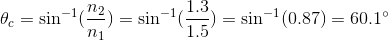# MCAT Physical : Snell's Law

## Example Questions

### Example Question #21 : Optics

A monochromatic light ray passes from air (n = 1.00) into glass (n = 1.50) at an angle of 20o with respect to the normal. What is the approximate angle of refraction?

17o

31o

27o

24o

13o

13o

Explanation:

To compare angles of incidence and refraction, use Snell's law.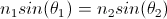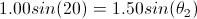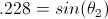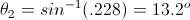Notice that as the light enters a more dense medium, it bends towards the normal.

### Example Question #22 : Optics

An incandescent light bulb is shown through a glass prism. The certain wavlength of the light is then directed into a glass cuvette containing an unknown concentration of protein. Commonly, this process is called spectroscopy and is used to determine the concentrations of DNA, RNA, and proteins in solutions. The indices of reflection of air, glass, and the solution are 1, 1.5, and 1.3, respectively.

The red light, selected for by the prism, is shown through air onto the glass cuvette at an angle of 30o to the normal. At what angle to normal does the light have when it is in the glass?

30o

45o

19.5o

22o

19.5o

Explanation:

This problem asks us to consider refraction, that is, the bending of light when it enters a new medium. Thinking back to our light formulas in physics, we know that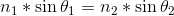, where n is the index of refraction of the medium and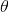is the angle the light ray makes to normal.

Using the information provided in the pre-question text and the question above, we can solve for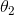.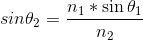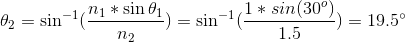### Example Question #23 : Optics

An incandescent light bulb is shown through a glass prism. The certain wavlength of the light is then directed into a glass cuvette containing an unknown concentration of protein. Commonly, this process is called spectroscopy and is used to determine the concentrations of DNA, RNA, and proteins in solutions. The indices of reflection of air, glass, and the solution are 1, 1.5, and 1.3, respectively.

Red light is selected for by the prism and shown onto the glass cuvette at an angle of 30o to the normal. At what angle to normal does the light travel when it is in the solution after it has moved through wall of the cuvette?

22.7o

45o

19.5o

35o

22.7o

Explanation:

Notice that, in this problem, there are two different changes in indices of refraction: air to glass and glass to solution; thus, we need to compute the angle change twice. First, let’s look at how the angle changes from air to glass. We know from Snell’s law that, where n is the index of refraction of the medium andis the angle the light ray makes to normal.

Rearranging, we can find the angle to normal in the glass.Now, we can use this angle and repeat the above equation to find the angle that the light enters the solution.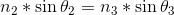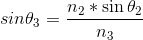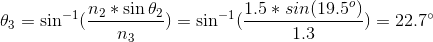### Example Question #24 : Optics

An incandescent light bulb is shown through a glass prism. The certain wavlength of the light is then directed into a glass cuvette containing an unknown concentration of protein. Commonly, this process is called spectroscopy and is used to determine the concentrations of DNA, RNA, and proteins in solutions. The indices of reflection of air, glass, and the solution are 1, 1.5, and 1.3, respectively.

If the solution had a higher protein concentration than expected, how would the angle of refraction change as light travels from the glass into the solution?

It would be larger or smaller, depending on the color of the light

It would remain the same

It would be larger

It would be smaller

It would be smaller

Explanation:

This question is asking us to consider what would happen if the solution were more concentrated, essentially, if there were more particles per unit volume (denser). If we think back to the definition of index of refraction, we know that it relates to the density of a medium. The denser the medium, the higher the index of refraction.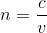Looking at Snell’s law, we can see the relationship between index of refraction and the angle of refraction.If the solution were more concentrated, n2 would increase, making the term on the right side of the equation smaller. The sin function of a smaller number gives a smaller angle; thus, as the concentration increases (and thus the index of refraction increases), the angle of refraction gets smaller.

### Example Question #31 : Optics

An incandescent light bulb is shown through a glass prism. The certain wavlength of the light is then directed into a glass cuvette containing an unknown concentration of protein. Commonly, this process is called spectroscopy and is used to determine the concentrations of DNA, RNA, and proteins in solutions. The indices of reflection of air, glass, and the solution are 1, 1.5, and 1.3, respectively.

At what angle would the light passing through the glass cuvette need to hit the solution inside the cuvette for no light to enter the solution?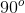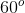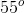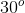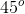Explanation:

First, we need to determine what this question is asking us to do. If no light entered the solution from the glass, we know this is total internal reflection. Remember that total internal reflection occurs when light from one medium hits a second medium at an angle higher that the critical angle. Thinking back to our physics formulas, we know that the critical angle can be determined by the equation below.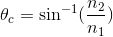Plugging in the values for glass and the solution, we can find the critical angle.